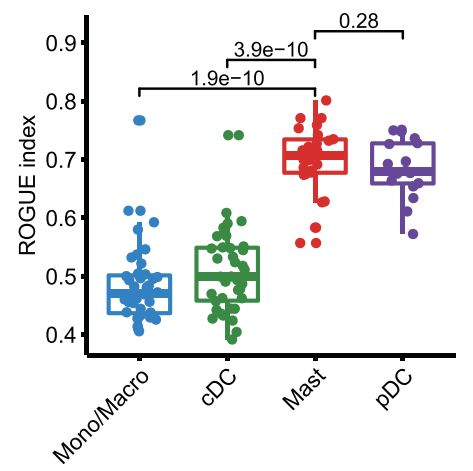# Using ROGUE to assessing the herterogeneity of single cell populations

#### Introduction

In this paper, by performing a pan-cancer analysis of single myeloid cells from 210 patients across 15 human cancer types, authors found that tumor-derived mast cells showed low expression of TNF in multiple cancer types. Apart from TNF, mast-derived VEGFA is a key gene associated with tumor angiogenesis.So they use ROGUE to assessing the herterogeneity of mast cells cluster in cancer.
ROGUE： Often, it is not even clear whether a given cluster is uniform in unsupervised scRNA-seq data analyses. Here, we proposed the concept of cluster purity and introduced a conceptually novel statistic, named ROGUE, to examine whether a given cluster is a pure cell population.

#### Code explanation

Load the required library and tool function. Single cell data in this research were processed for dimension reduction and unsupervised clustering by following the workflow in Scanpy, so the result of Scanpy(h5ad files) were read to run ROGUE.

``````.libPaths("/data2/csj/tools/Rlib3.6")
suppressMessages(library(ROGUE))
suppressMessages(library(ggplot2))
suppressMessages(library(tidyverse))

library(dplyr)
library(monocle)
options(stringsAsFactors=FALSE)
library(reticulate)

parse_h5ad <- function(adata){
require(reticulate)
ad <- import("anndata", convert = FALSE)
ada <- ad\$read_h5ad(adata)
meta <- py_to_r(ada\$obs)
if(class(ada\$raw\$X) == "scipy.sparse.csr.csr_matrix"){
exp <- t(py_to_r(ada\$raw\$X\$toarray()))
}
else{
exp <- t(py_to_r(ada\$raw\$X))
}
rownames(exp) <- rownames(py_to_r(ada\$raw\$var))
colnames(exp) <- rownames(meta)
return(
list(
metadata = meta,
expression = exp
)
)
}
``````

read h5ad data. Extracted expresion matrix, cluster name, sample name from h5ad

``````
### using the merged dataset of A20191105
h5ad_label <- parse_h5ad("/data2/csj/Pan_Myeloid/A20191105/scanorama_integrate/All_Myeloid.h5ad")
cancers <- c("ESCA","L", "MM", "OV", "PACA", "RC", "THCA", "UCEC")
meta_merged <- h5ad_label\$metadata
meta_merged <- meta_merged[meta_merged\$cancer %in% cancers,]
meta_merged <- meta_merged[rownames(meta_merged) %in% rownames(meta),]

expr <- expr[,rownames(meta_merged)]
meta <- meta_merged
meta\$M1 <- unlist(lapply(as.vector(meta\$MajorCluster),function(x){unlist(strsplit(x,"_"))}))

current.cluster.ids <- c("Monolike","Macro","cDC2","Mast","cDC1","pDC","cDC3", "Mono")
new.cluster.ids <- c("Mono/Mq","Mono/Mq","cDC2","Mast","cDC1","pDC","cDC3", "Mono/Mq")
meta\$M_cluster <- plyr::mapvalues(x = meta\$M1, from = current.cluster.ids, to = new.cluster.ids)
``````

Run rogue

``````rogue.res <- rogue(expr, labels = meta\$M_cluster, samples = meta\$patient, platform = "UMI", span = 0.6)
rogue.boxplot(rogue.res)
``````

Authors used a major cell classes this time.

``````current.cluster.ids <- c("Monolike","Macro","cDC2","Mast","cDC1","pDC","cDC3", "Mono")
new.cluster.ids <- c("Mono/Mq","Mono/Mq","cDC","Mast","cDC","pDC","cDC", "Mono/Mq")
meta\$MM_cluster <- plyr::mapvalues(x = meta\$M1, from = current.cluster.ids, to = new.cluster.ids)``````

And run rogue again

``````res <- rogue(expr, labels = meta\$MM_cluster, samples = meta\$patient, platform = "UMI", span = 0.6)
rogue.boxplot(res)

saveRDS(rogue.res,"Sub_ROGUE.rds")
saveRDS(res,"/data2/csj/Pan_Myeloid/Maj_ROGUE.rds")
``````

Plot figure to compare ROGUE index between different clusters.

``````res <- readRDS("/data2/csj/Pan_Myeloid/Sub_ROGUE.rds")
res\$patient <- rownames(res)
df <- melt(res)
df <- df[df\$variable %in% c('pDC','cDC1','cDC2','cDC3'),]
# df\$variable <- factor(df\$variable, levels=c("Mono/Mq","cDC1","cDC2","cDC3","pDC","Mast"))
df\$variable <- factor(df\$variable, levels=c("pDC","cDC1","cDC2","cDC3"))

ggboxplot(df, x = "variable", y = "value",color = "variable",palette ='Paired',
add = c("mean_se", "dotplot"))+theme(legend.position='none')+ylab("ROGUE index")+xlab("")

ggboxplot(df, x = "variable", y = "value",color = "variable",palette ='Paired',
ylab = "ROGUE index",
add = "jitter",                              # Add jittered points
add.params = list(size = 1.5, jitter = 0.2)  # Point size and the amount of jittering
)+theme(legend.position='none')
``````

Use different color panels, and stat_compare_means function to make test

``````# Color panel -----------------
c54 <- c("#1A62AF","#26933B","#F3951B","#E71638")
my_comparisons <- list( c("cDC2", "pDC"), c("cDC2", "cDC1"), c("cDC2", "cDC3") )
p <- ggboxplot(df, x = "variable", y = "value",color = "variable", palette = c54,
ylab = "ROGUE index",
add = "jitter",                              # Add jittered points
add.params = list(size = 1.5, jitter = 0.2)  # Point size and the amount of jittering
)+theme(legend.position='none')+stat_compare_means(comparisons = my_comparisons)+theme(
legend.position = "null",
axis.text.x = element_text(
size = 12,
angle = 45,
hjust = 1
))
pdf("/data2/csj/Pan_Myeloid/A20191105/ROGUE_cDC.pdf",width=3.41,height=3.56)
p
dev.off()

library(ggbeeswarm)
ggplot(df, aes_string(x = "variable", y = "value")) +
theme_bw() + xlab("") + ggtitle("") + ylab("ROGUE index")+
theme(
legend.position = "null",
plot.title = element_text(
size = 16,
face = "bold",
hjust = 0.5
),
text = element_text(size = 10),
plot.margin = unit(c(1, 1, 1, 1), "char"),
axis.text.x = element_text(
size = 12,
angle = 45,
hjust = 1
),
axis.text.y = element_text(size = 12),
axis.title = element_text(size = 15)
)+ geom_boxplot(
outlier.alpha = 0.5,
outlier.color = "#a3a3a3",
outlier.size = 2
) + geom_quasirandom(
cex = 2,
aes_string(color="variable"),
width = 0.25,
alpha = 1
) + scale_color_manual(values = c54)

res <- readRDS("/data2/csj/Pan_Myeloid/Maj_ROGUE.rds")
res\$patient <- rownames(res)
df <- melt(res)

my_comparisons <- list( c("Mast", "Mono/Mq"), c("Mast", "cDC"), c("Mast", "pDC") )
p <- ggboxplot(df, x = "variable", y = "value",color = "variable", palette = c('dodgerblue2','green4','#E31A1C','#6A3D9A'),
ylab = "ROGUE index",
add = "jitter",                              # Add jittered points
add.params = list(size = 1.5, jitter = 0.2)  # Point size and the amount of jittering
)+theme(legend.position='none')+stat_compare_means(comparisons = my_comparisons)+theme(
legend.position = "null",
axis.text.x = element_text(
size = 12,
angle = 45,
hjust = 1
))
pdf("/data2/csj/Pan_Myeloid/A20191105/ROGUE_major.pdf",width=3.41,height=3.56)
p
dev.off()

# Color panel -----------------
c54 <- c('dodgerblue2','green4','#E31A1C','#6A3D9A','#FF7F00',
'#FB9A99','#CAB2D6','khaki2','deeppink1','blue1',
'steelblue4','green1','yellow4','yellow3','forestgreen',
'red2','orange','cornflowerblue', 'magenta','darkolivegreen4',
'indianred1','tan4','darkblue','mediumorchid1','firebrick4',
'yellowgreen','lightsalmon','tan3','tan1','darkgray',
'wheat4','#DDAD4B','chartreuse','seagreen1','moccasin',
'mediumvioletred','seagreen','cadetblue1','darkolivegreen1','tan2',
'tomato3','#7CE3D8','gainsboro','gold1','skyblue2',
'palegreen2','#FDBF6F','gray70','darkorange4','orchid1',
'darkturquoise','maroon','brown','black')
library(ggbeeswarm)
ggplot(df, aes_string(x = "variable", y = "value")) +
theme_bw() + xlab("") + ggtitle("") + ylab("ROGUE index")+
theme(
legend.position = "null",
plot.title = element_text(
size = 16,
face = "bold",
hjust = 0.5
),
text = element_text(size = 10),
plot.margin = unit(c(1, 1, 1, 1), "char"),
axis.text.x = element_text(
size = 12,
angle = 45,
hjust = 1
),
axis.text.y = element_text(size = 12),
axis.title = element_text(size = 15)
)+ geom_boxplot(
outlier.alpha = 0.5,
outlier.color = "#a3a3a3",
outlier.size = 1
) + geom_quasirandom(
cex = 1,
aes_string(color="variable"),
width = 0.25,
alpha = 1
) + scale_color_manual(values = c54)``````#### Interpretation of results

Mast cells showed the highest homogeneity among the four major lineages, so is is possibly a cluster that cannot be subdivided. Then authors directly compared the expression patterns of TNF and
VEGFA in mast cells and observed both mutually exclusive
and co-expression pattern across different cancer types

#### References

Cheng S , Li Z , Gao R , et al. A pan-cancer single-cell transcriptional atlas of tumor infiltrating myeloid cells[J]. Cell, 2021, 184(3):792-809.e23.
ROGUE package: https://github.com/PaulingLiu/ROGUE

#### Original

https://github.com/Sijin-ZhangLab/PanMyeloid